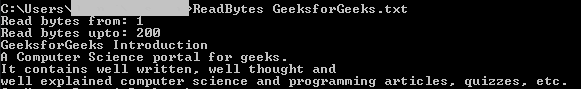Related Articles

# C program to read a range of bytes from file and print it to console

• Last Updated : 28 Jun, 2021

Given a file F, the task is to write C program to print any range of bytes from the given file and print it to a console.

Functions Used:

1. fopen(): Creation of a new file. The file is opened with attributes as “a” or “a+” or “w” or “w++”.
2. fgetc(): Reading the characters from the file.
3. fclose(): For closing a file.

Approach:

• Initialize a file pointer, say File *fptr1.
• Initialize an array to store the bytes that will be read from the file.
• Open the file using the function fopen() as fptr1 = fopen(argv, “r”).
• Iterate a loop until the given file is read and stored, the characters are scanned in the variable, say C using the fgetc() function.
• Store each character C extracted in the above step, to a new string S and print that string using the printf() function.
• After completing the above steps, close the file using the fclose() function.

Below is the implementation of the above approach:

## C

 `// C program to read particular bytes``// from the existing file``#include ``#include `` ` `// Maximum range of bytes``#define MAX 1000`` ` `// Filename given as the command``// line argument``int` `main(``int` `argc, ``char``* argv[])``{``    ``// Pointer to the file to be``    ``// read from``    ``FILE``* fptr1;``    ``char` `c;`` ` `    ``// Stores the bytes to read``    ``char` `str[MAX];``    ``int` `i = 0, j, from, to;`` ` `    ``// If the file exists and has``    ``// read permission``    ``fptr1 = ``fopen``(argv, ``"r"``);`` ` `    ``if` `(fptr1 == NULL) {``        ``return` `1;``    ``}`` ` `    ``// Input from the user range of``    ``// bytes inclusive of from and to``    ``printf``(``"Read bytes from: "``);``    ``scanf``(``"%d"``, &from);``    ``printf``(``"Read bytes upto: "``);``    ``scanf``(``"%d"``, &to);`` ` `    ``// Loop to read required byte``    ``// of file``    ``for` `(i = 0, j = 0; i <= to``                       ``&& c != EOF;``         ``i++) {`` ` `        ``// Skip the bytes not required``        ``if` `(i >= from) {``            ``str[j] = c;``            ``j++;``        ``}`` ` `        ``// Get the characters``        ``c = ``fgetc``(fptr1);``    ``}`` ` `    ``// Print the bytes as string``    ``printf``(``"%s"``, str);`` ` `    ``// Close the file``    ``fclose``(fptr1);`` ` `    ``return` `0;``}`

Output:Want to learn from the best curated videos and practice problems, check out the C Foundation Course for Basic to Advanced C.

My Personal Notes arrow_drop_up